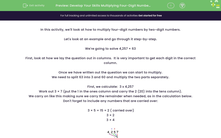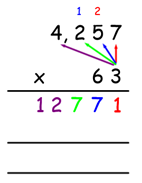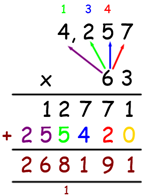# Develop Your Skills Multiplying Four-Digit Numbers by Two-Digit Numbers

In this worksheet, students will learn how to multiply four-digit numbers by two-digit numbers.Key stage:  KS 2

Curriculum topic:   Number: Addition, Subtraction, Multiplication and Division

Curriculum subtopic:   Multiply to Four Digits (Long Multiplication)

Difficulty level:#### Worksheet Overview

In this activity, we'll look at how to multiply four-digit numbers by two-digit numbers.

Let's look at an example and go through it step-by-step.

We're going to solve 4,257 × 63

First, look at how we lay the question out in columns.  It is very important to get each digit in the correct column.

Once we have written out the question we can start to multiply.

We need to split 63 into 3 and 60 and multiply the two parts separately.

First, we calculate:  3 x 4,257

Work out 3 × 7 (put the 1 in the ones column and carry the 2 (20) into the tens column).

We carry on like this making sure we carry the remainder when needed, as in the calculation below.

Don't forget to include any numbers that are carried over:

3 × 5 = 15 + 2 ( carried over)

3 × 2

3 × 4So, we now know that 4,257 x 3 = 12,771

Now we calculate:  6 (60) x 4,257First, we put a zero as a placeholder in the ones column. Can you see it in yellow in the calculation above?

Now, we multiply as we did before, carrying where needed.

6 × 7

6 × 5

6 × 2

6 × 4

We must remember to add on the numbers that we carry over.

This gives us 255,420

Finally, add the two numbers together: 12,771 + 255,420 = 268,191

Top Tips:

Lay out the question in columns - squared paper can help.

Remember to put a  zero as a placeholder.

Remember to carry over when needed.

Add on any digits that are carried over.

Phew! That was a lot to do.

Now, let's put this method to the test!

Work through each question one step at a time and if you need to look at this example again, simply click on the red help button on the screen.### What is EdPlace?

We're your National Curriculum aligned online education content provider helping each child succeed in English, maths and science from year 1 to GCSE. With an EdPlace account you’ll be able to track and measure progress, helping each child achieve their best. We build confidence and attainment by personalising each child’s learning at a level that suits them.

Get started••••